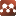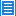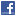## Nuclear and Atomic Physics## Beta Decay Emits No Neutrino

Authors: Chan Rasjid Kah Chew

The 1927 Ellis-Wooster calorimetry experiment was an attempt to resolve the controversy over the continuous energy distribution spectrum of beta decay. A Radium E source was placed within a calorimeter in order to capture and measure the heat generated by beta decay. If the beta decay energy is assumed to be quantized, the captured heat energy should match the maximum spectrum energy of 1.05 MeV if the calorimeter captured all the disintegration energy. The result of the experiment gave the captured average heat of beta decay to be 350,000 eV instead of the expected 1.05 MeV. The 350,000 eV was accepted to be a match to the average spectrum energy of 390,000 eV. The experiment indicated some energy escaped the Ellis-Wooster calorimeter - thus the notion of "missing energy". The thesis of this paper is that the conclusion of the Ellis-Wooster experiment depends on whether the heat of calorimetry is consistent with relativistic kinetic energy or with classical kinetic energy. The spectrum energy used by the experiment was based on relativistic energy. If the values are converted to classical energy, the the maximum spectrum energy would only be 230,000 eV and the average 120,000 eV. The captured heat was much greater than the average of 120,000 eV. This reinterpretation would dismiss the notion of any missing energy in the experiment. The question of whether there was any missing energy is related to whether physical reality is consistent with special relativity or with Newtonian mechanics. The basis upon which Wolfgang Pauli proposed his 1930 neutrino hypothesis was the conclusion of the 1927 Ellis-Wooster experiment which supposedly supported the idea of "missing energy". The neutrino and the current neutrino physics would remain if special relativity is found to be the correct mechanics representing the physical world. On the other hand, if Newtonian mechanics is found to be correct, then all of neutrino physics would have to be dismissed. The one experiment that could decide on the issue is to determine the maximum speed with which beta particles are ejected in beta decay using the direct time-of-flight method. If Newtonian mechanics is correct, then there would be beta particles found to go beyond the speed of light; otherwise, it would be experimental evidence supporting special relativity. The result of this experiment would settle unequivocally the question concerning the nature of physical reality. But to date, this experiment has not been carried out.